• 通俗来讲，一维傅里叶变换是将一个一维的信号分解成若干个三角波。 对于一个三角波而言，需要三个参数来确定它：频率,幅度 A ，相位。因此在频域中，一维坐标代表频率，而每个坐标对应的函数值也就是是一个复数，...
• 本程序主要实现了二维傅里叶变换，其中先对图像矩阵进行预处理（即图像中心化），然后进行行傅里叶变换，再对其进行列变换，进行行列变换是调用自己写的一维傅里叶变换函数ImFFT实现的。程序输入为图像矩阵A，输出为...
• 资源为二维傅里叶变换的C++实现，内含多个示例，比较详细。
• 图像的二维傅里叶变换频谱图特点研究，以及基于halcon得到的一些图谱分析。
• 二维傅里叶变换 一个随时间或空间变化的周期函数（信号）f(x),可以看作是许多具有不同频率的基元傅里叶变换
• 提出了合成孔径激光成像雷达（SAIL）的二维傅里叶变换成像算法，即对回波信号进行顺轨向相位二次项共轭补偿后直接实施二维傅里叶变换。归纳了啁啾光源侧视SAIL，平移二次项波面直视SAIL和偏转平面波面直视SAIL的数据...
• 主要介绍了C++实现二维图形的傅里叶变换的方法,是C++程序设计里一个重要的应用,需要的朋友可以参考下
• 二维快速傅里叶变换-C语言-迭代法.c 实现方法为：C语言，先对每一行做傅里叶，再对结果的每一列做傅里叶
• 针对大口径光学元件干涉测试过程中,测试装置和干涉腔长较大,气流扰动和环境振动对移相测试过程产生影响等问题,采用一种基于二维傅里叶变换的单帧干涉图处理方法,只需要对一幅空间载频干涉条纹图进行处理即可获得待测...
• % 对中心化变换后的图像求二维傅里叶变换 figure,imshow(imt_f,[]); title('使用自编函数fftcenter()中心变换图的二维傅里叶图谱'); mod_imt = abs(imt_f); % 要求幅度谱，需对傅里叶变换求模 mod_imt_log = uint8...

function imt = fftcenter(ima) % 图像中心化转换函数

[xs,ys] = size(ima);

for i = 1:xs

for j = 1:ys

imt(i,j) = ima(i,j)*power((-1),(i+j)) ;%输入图像每个像素点乘以(-1)^x+y因子

end

end

return

%%%%%%%%%%%%%%%%%%%%%%%%%%%%%%%%%%%%%

% 2-D FFT 程序包

%%%%%%%%%%%%%%%%%%%%%%%%%%%%%%%%%%%%%

function proj04();

imshow(ima);

title('原始图像');

ima_f = fft2(ima); % 对图像ima求二维快速傅里叶变换

mod_ima = abs(ima_f) % 要求幅度谱，需对傅里叶变换求模

mod_ima_log = uint8(log2((mod_ima))) % 要求幅度谱需对FFT后得到的模取对数

phase_ima = atan2(imag(ima_f),real(ima_f)); % 求相位谱，arctan必须使用一个四象限反正切来计算，调用MATLAB的atan2函数

figure,subplot(1,2,1),imshow(mod_ima_log,[]); % []的作用是将mod_ima_log的最大最小值分别作为纯白255和纯黑0，中间值映射到0到255之间

title('输入图像的二维傅里叶幅度谱');

subplot(1,2,2),imshow(phase_ima,[]);

title('输入图像的二维傅里叶相位谱');

threeD_mod_ima_log = log2(mod_ima)+1;

[x1,y1] = size(threeD_mod_ima_log);

[a1,b1]=meshgrid(1:x1,1:y1); % 使用函数mesh绘制三维傅立叶图需要先进行meshgrid

figure,subplot(1,2,1),mesh(a1,b1,threeD_mod_ima_log);

title('输入图像的三维傅里叶幅度谱');

[x2,y2] = size(phase_ima);

[a2,b2] = meshgrid(1:x2,1:y2); % 使用函数mesh绘制三维傅立叶图需要先进行meshgrid

subplot(1,2,2),mesh(a2,b2,phase_ima);

title('输入图像的三维傅里叶相位谱');

%

s = fftshift(ima_f); % 调用fftcenter函数实现图像的中心化变换(为了对比fftcenter)

mod_s = abs(s) % 要求幅度谱，需对傅里叶变换求模

mod_s_log = uint8(log2(mod_s)) % 要求幅度谱需对FFT后得到的模取对数

phase_s = atan2(imag(s),real(s)); % 求相位谱，arctan必须使用一个四象限反正切来计算，调用MATLAB的atan2函数

figure,imshow(mod_s_log,[]); % []的作用是将mod_ima_log的最大最小值分别作为纯白255和纯黑0，中间值映射到0到255之间

title('fftshift函数的中心变换图的二维傅里叶幅度谱');

imt = fftcenter(ima); % 调用自编函数对图像进行中心化变换

imt_f = fft2(imt); % 对中心化变换后的图像求二维傅里叶变换

figure,imshow(imt_f,[]);

title('使用自编函数fftcenter()中心变换图的二维傅里叶图谱');

mod_imt = abs(imt_f); % 要求幅度谱，需对傅里叶变换求模

mod_imt_log = uint8(log2(mod_imt)); % 要求幅度谱需对FFT后得到的模取对数

phase_imt = atan2(imag(imt_f),real(imt_f)); % 求相位谱，arctan必须使用一个四象限反正切来计算，调用MATLAB的atan2函数

figure,subplot(1,2,1),imshow(mod_imt_log,[ ]);

title('中心变换图的二维傅里叶幅度谱');

subplot(1,2,2),imshow(phase_imt,[]),

title('中心变换图的二维傅里叶相位谱');

%

threeD_mod_imt_log = log10(mod_imt)+1;

[x3,y3] = size(threeD_mod_imt_log);

[a3,b3]=meshgrid(1:x3,1:y3); % 使用函数mesh绘制三维傅立叶图需要先进行meshgrid

figure,subplot(1,2,1),mesh(a3,b3,threeD_mod_imt_log);

title('中心变换图的三维傅里叶幅度谱');

[x4,y4] = size(phase_imt);

[a4,b4] = meshgrid(1:x4,1:y4); % 使用函数mesh绘制三维傅立叶图需要先进行meshgrid

subplot(1,2,2),mesh(a4,b4,phase_imt);

title('中心变换图的三维傅里叶相位谱');

%

imt_if = ifft2(imt_f);

imt_if_abs = abs(imt_if);

figure,subplot(1,2,1),imshow(imt_if_abs,[]);

title('中心化变化图像');

subplot(1,2,2),imshow(ima);

title('原始图像');

%%%%%%%%%%%%%%%%%%%%%%%%%%%%%%%%%%%%

% 设计一个高斯滤波器

%%%%%%%%%%%%%%%%%%%%%%%%%%%%%%%%%%%%

function im=Lowpassfiltering()

[M,N] = size(st);

D0 = 20; % 设定截止频率D0

x = 2*M;

y = 2*N;

% 进行高斯滤波之前先对图像进行填充，填充后的图像尺寸为(2M*2N)

for i=1:x

for j=1:y

if((i<=M)&&(j<=N))

st(i,j)=st(i,j);

else

st(i,j)=0;

end

end

end

figure,imshow(st);

title('对输入图像进行填充');

imwrite(st,'K1.jpg');

figure(2);

st = fftshift(fft2(st)); % 自编函数中心化效果有问题，因此调用fftshift函数实现中心化

for i = 1:x

for j = 1:y

D(i,j) = sqrt((i-M)^2+(j-N)^2); % 中心点为(M,N)

h(i,j) = 1*exp(-1/2*(D(i,j)^2/D0^2)); % 系统函数H(u,v)

st(i,j) = h(i,j)*st(i,j); % 滤波过程

end

end

st = fftshift(ifft2(st)); % 进行傅里叶反变换时再进行一次中心化

st_abs = abs(st);

for i=1:M

for j=1:N

st_k(i,j)=st_abs(i,j); % 对图像进行裁剪，去除填充部分

end

end

imshow(st_k,[])

title('GLPF滤波后的输出图像(已裁剪)');

for i=1:M

for j=1:N

st_k2(i,j) = st_k1(i,j) - ( st_k(i,j)); % 原始图像减去GLPF图像，得到锐化图像

end

end

figure,imshow(st_k2,[])

title('原始图像减去GLPF滤波图像后的锐化图像');

展开全文• 回顾一下一维FT公式： 通俗来讲，一维傅里叶变换是将一个一维的信号分解成若干个复指数波。而由于 ，所以可以将每一个复指数波 都视为是 余弦波+j*正弦波的组合。对于一个正弦波而言，需要三...

点击上方“机器学习与生成对抗网络”，关注"星标"

获取有趣、好玩的前沿干货！

来自 | 知乎  阿姆斯特朗

链接 | https://zhuanlan.zhihu.com/p/110026009

文仅交流，侵删

1.回顾一下一维FT

公式：通俗来讲，一维傅里叶变换是将一个一维的信号分解成若干个复指数波。而由于，所以可以将每一个复指数波都视为是

余弦波+j*正弦波的组合。

对于一个正弦波而言，需要三个参数来确定它：频率,幅度，相位。因此在频域中，一维坐标代表频率，而每个坐标对应的函数值也就是是一个复数，其中它的幅度就是这个频率正弦波的幅度，相位就是。下图右侧展现的只是幅度图，在信号处理中用到更多的也是幅度图。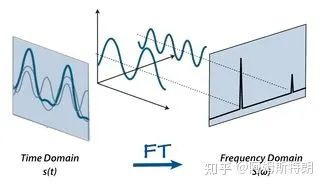一维傅里叶变换就是一个基变换，在时域中，基是一族冲激信号,在频域中；基是，而且这组基是正交基。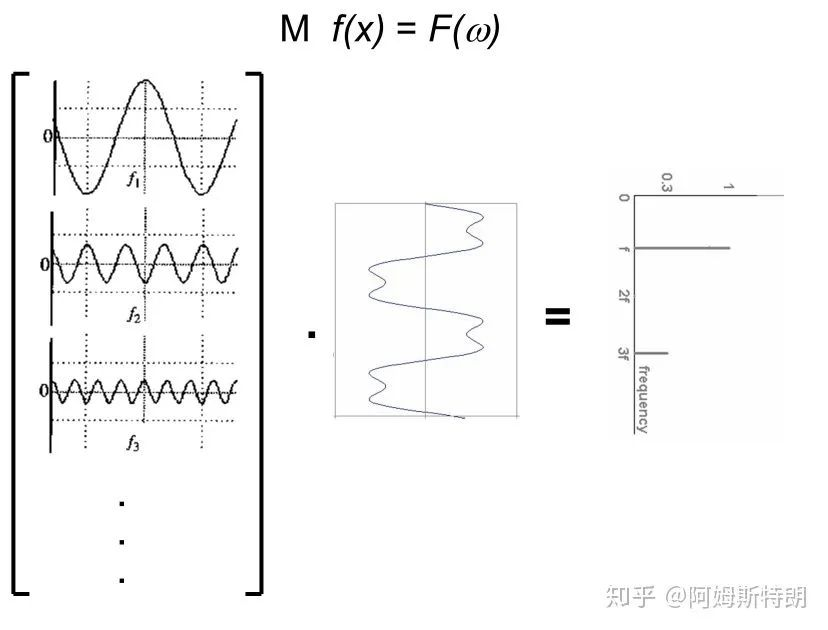F=Mf，基变换示意图

2.类比：从一维到二维

一维信号是一个序列，FT将其分解成若干个一维的简单函数之和。二维的信号可以说是一个图像，类比一维，那二维FT是不是将一个图像分解成若干个简单的图像呢？

确实是这样，

二维FT将一个图像分解成若干个复平面波之和。如下图：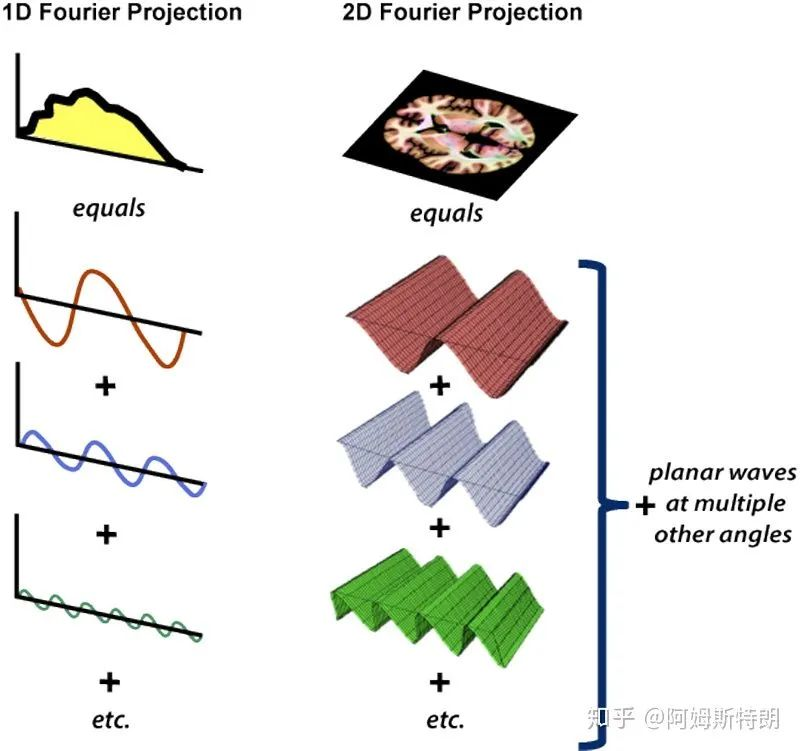二维FT的公式:通过公式，我们可以计算出，

每个平面波在图像中成分是多少。从公式也可以看到，二维傅里叶变换就是将图像与每个不同频率的不同方向的复平面波做内积(先点乘在求和)，也就是一个

求在基上的

投影的过程。(应该知道是b在a上的投影，只不过这里的|a|的值被设为1，所以只有内积)

3.什么是二维频率域K-SPACE

对于正弦平面波，可以这样理解，在一个方向上存在一个正弦函数，在法线方向上将其拉伸。前面说过三个参数可以确定一个一维的正弦波。哪几个参数可以确定一个二维的正弦平面波呢？答案是四个，其中三个和一维的情况一样(频率,幅度，相位)，但是具有相同这些参数的平面波却可以有不同的方向。如下图所示：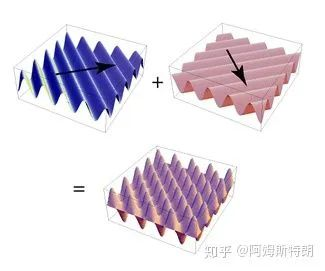两个不同方向的平面波叠加

类比一维中，幅度和相位可以用一个复数表示，它可以作为我们存储的内容。但是还有两个：一个频率一个方向。这时想到向量是有方向的，也是有长度的。所以我们用一个二维的矩阵的来保存分解之后得到的信息。这个矩阵就是K空间。(一般用k来表示空间频率，单位是1/m)

什么意思呢？就是说一个二维矩阵点代表这个平面波的法向量，这个向量的模代表这个平面波的频率，这个点里面保存的内容复数就是此平面波的幅度和相位。下面这个图很好的体现了这一点：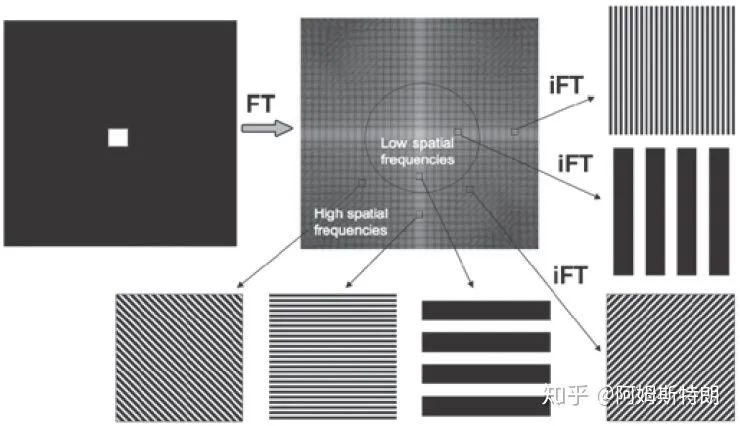也因此K空间的中心对于低频，周围对于高频。如下图，K空间中只有(0，0)处有值，也就是信号都是直流即不存在变化，所以实空间就是一张白纸。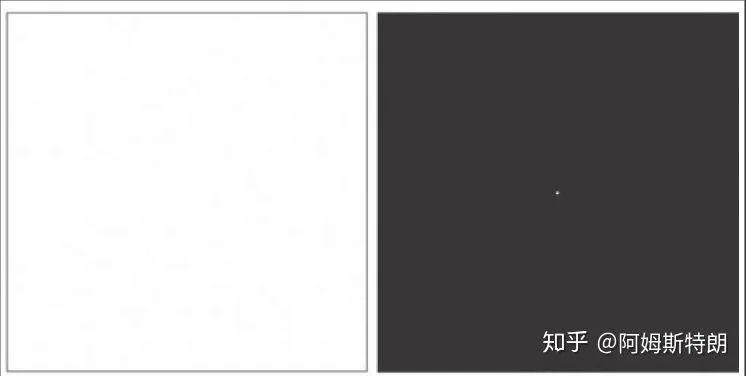再如下面这个图片，

中心低频贡献了图像的主体，周围高频提供图像的细节和边缘。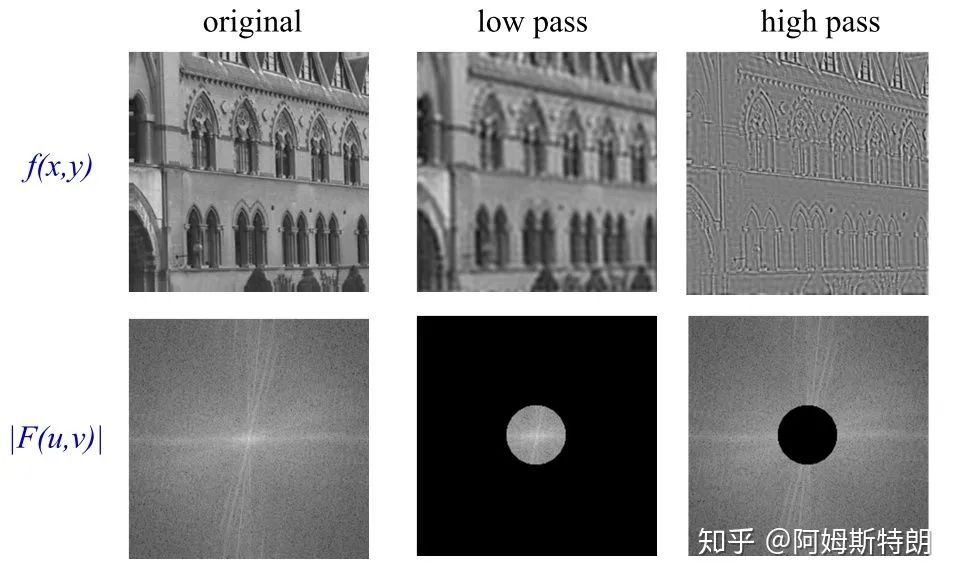因此，k空间的每一个位置存储的数代表了所在位置复平面波在图像中占多少成分，我们就可以用

每个系数*所代表的平面波相加得到原来的图像，也就是下图。

所以k空间和对应图像储存的信息含量是一样的，只不过表现形式不同，或者说基不同。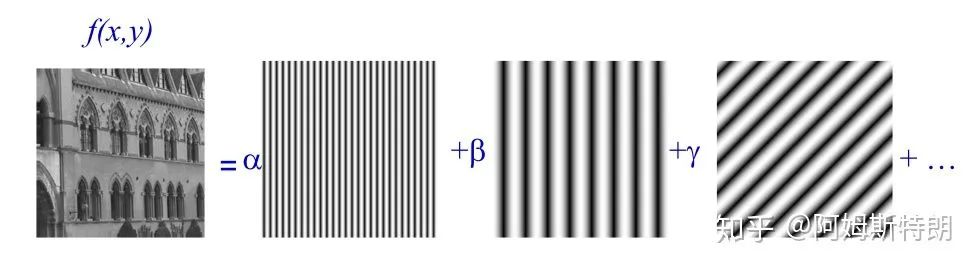4.K空间的一些性质

离散的2D-FT

在数字图像中，数据都是离散的。也就涉及到采样的问题，和一维一样，如果采样率过低，k空间就会混叠。同时在k空间中采样过低，图像也会混叠。

FOV和分辨率在k空间和图像中是相反的关系。也就是：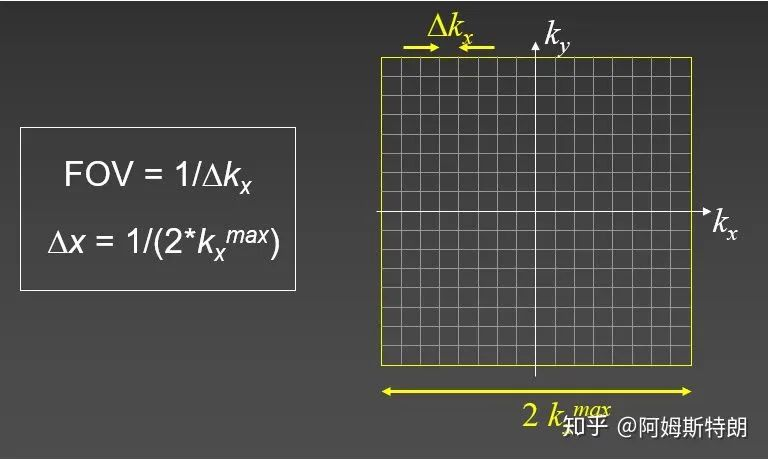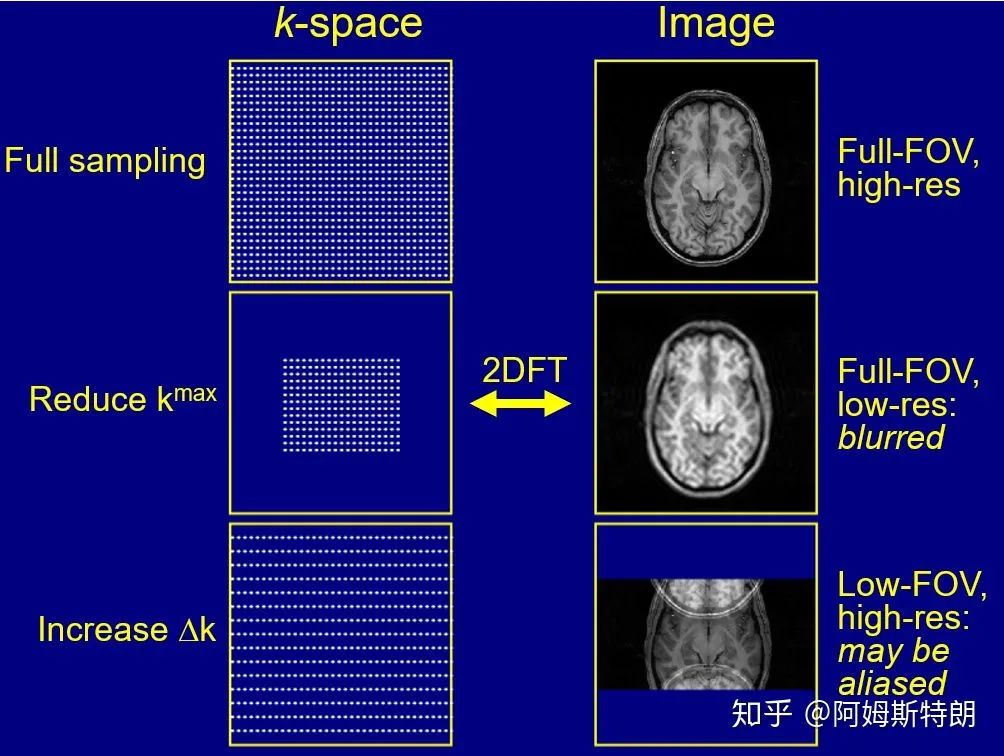减小kmax，使得图像分辨率下降，减小Δk，使得FOV缩小。

旋转不变性

从平面波的角度很容易理解，旋转没有改变平面波的幅度相位，只是将所有的平面波都旋转了一个角度。下面这个图像显示了二维傅里叶变换中，实空间旋转多少，频率空间也会相应旋转多少。这其实是

高维傅里叶变换缩放定理的一种特殊情况。(连续的是可以证明的，离散的涉及插值 ，不一定完全准确)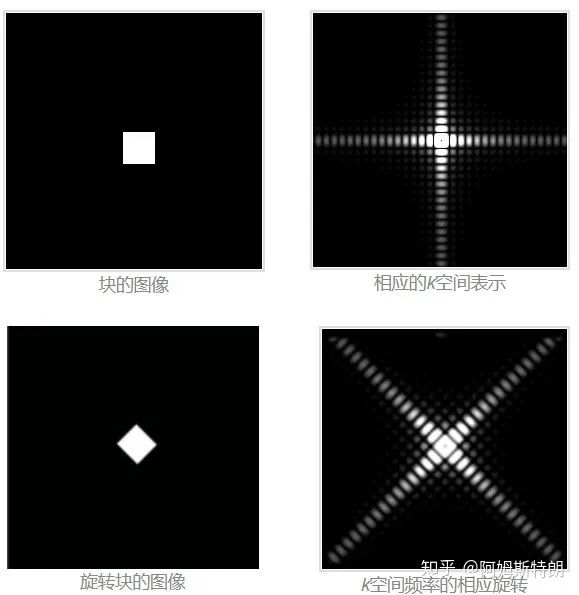5.其他

1.因为matlab中的fft算法都是将0放在第一个的，所有写matlab时一定要将k空间fftshift一下使得零频回到k空间中心。

2.简单的应用k空间进行去噪例子。通过去掉明显的k空间的异常峰，可以去除图像中有规律变化的噪声或者伪影。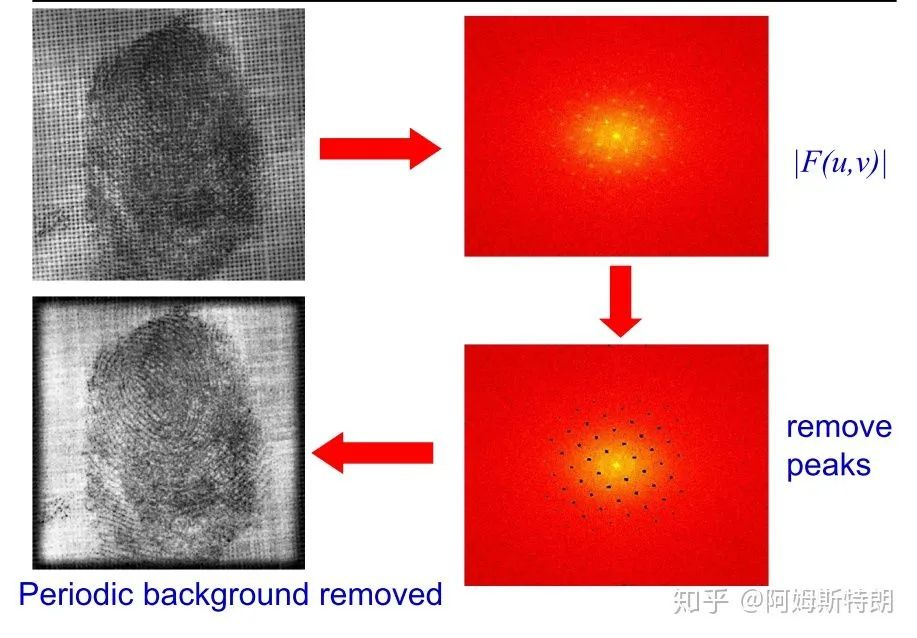指纹去噪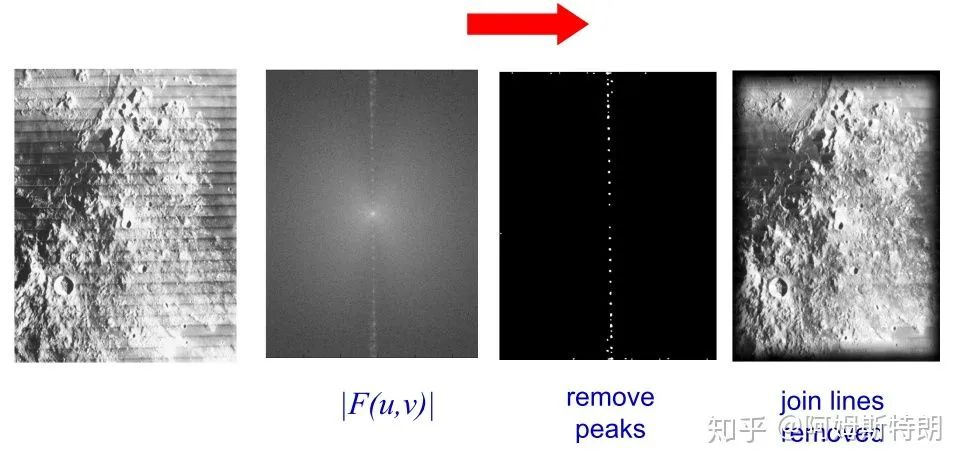天体表面去噪

6、评论及解答

1、始终想不通为啥各个波的叠加能反应出某一个像素点的信号大小

你把它理解向量分解就懂了，平面波就是基，k空间里的数就是基的系数。你得到系数(k空间)的时候就是在投影，变为原向量(图像)就是叠加。只不过这里一个图像是一个向量而已。

复平面波叠加时，先经过放大(幅度)在经过移位(相位)，相位信息里保留了很多位置信息，可以查看我的另一个回答：

为什么用图像二维傅里叶变换的相位谱进行反变换，能够大致得到原图的形状，而幅度谱则不行呢？

2、傅里叶变换后图像是关于频率矩形中心对称的，那么对称的4个平面三角波不就是注定了幅值和相角，频率是相等的。那么这样不是说明了任何波都是包含了4个这样对称的三角波，由它们组成？

是中心共轭对称，而没有左右上下的对称。而且只有实数图像的k空间才有这种特点，这是为了将复平面波中的虚部抵消掉，只留下实数部分。复数图像的k空间没有共轭对称的特点。下面是k空间的一部分数据，中心点为(101，101)。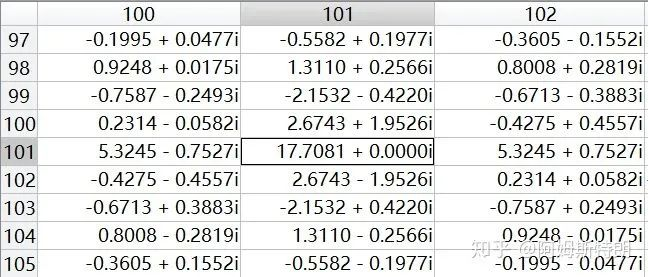参考：

 http://mriquestions.com

 A. Zisserman's lecture in B14 Image Analysis

链接：http://www.robots.ox.ac.uk/~az/lectures/ia/lect2.pdf

(这个slides真的不错，大家可以下载看看，里面也有其他的内容)

展开全文• 资源中包含了用matlab编写的二维快速傅里叶变换函数源代码，调用格式如下： y=myfft2(x);
• 傅里叶级数、一维傅里叶变换到二维傅里叶变换数理推导 参考资料： 如何理解傅里叶级数公式 从傅里叶级数到傅里叶变换 高维傅里叶变换的推导 IDL实现傅里叶变换 傅里叶级数 一维傅里叶变换 二维傅里叶变换 ...

傅里叶级数、一维傅里叶变换到二维傅里叶变换数理推导

想要用傅里叶变换的思维处理一个时序数据，将时序信息转换为参数信息，实现降维的目的。大致了解了一下傅里叶级数，如何从傅里叶级数转到傅里叶变换以及一维傅里叶变换和二维傅里叶变换的过渡，傅里叶变换的求解，快速傅里叶变换等知识。
傅里叶变换是一种从时域信息转为频域信息的手段，可以理解为从不同的角度来观察一个事物，例如总是被用来举例的听到的钢琴曲和乐谱，就是一个是时域一个频域，但是无论是时间维度听到的钢琴声还是频域维度看到的乐谱，他俩实质上描述的东西是一样的。

傅里叶级数

首先是傅里叶级数的概念以及这个概念被提出来的初衷。
最早，数学家发现某些周期函数可以由三角函数的和来表示，后来又有人猜测任意周期函数都可以写为三角函数之和。只需要三角函数之和函数满足以下几点：1）有常数项；2）同时包含奇函数和偶函数来组合出任意函数；3）周期与原函数周期相同，为T;4)可以调整振幅来逼近原函数（也就是系数项）。
构造出来的三角函数之和函数大致如下：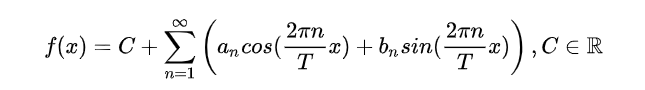接下来的重点是如何来确定参数：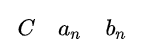在确定参量的时候，引入欧拉公式：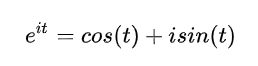最终得到的系数表达式为：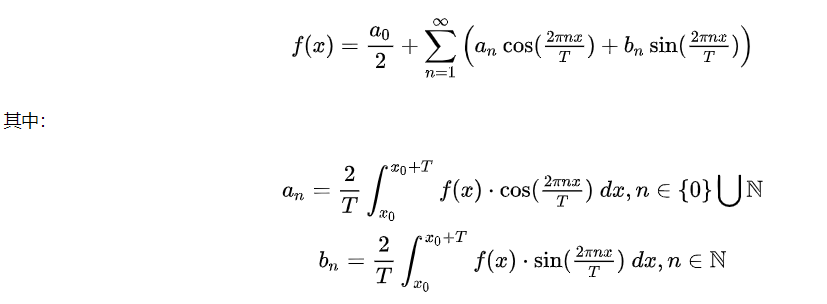具体的函数构造以及求解原理可以参照博客如何理解傅里叶级数公式

傅里叶变换

傅立叶级数是基于周期函数的，如果我们把周期推广到无穷，那么也就变为了非周期函数，这就是傅立叶变换。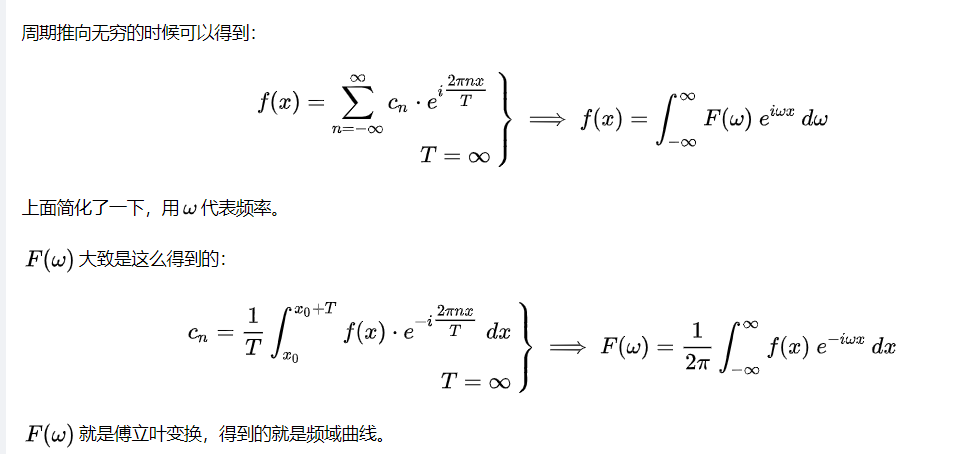一维傅里叶变换到二维傅里叶变换

对于傅里叶变换从一维到二维的扩展，主要参照知乎的一个回答
类比于一维傅里叶变换将一个函数分解成若干个一维的简单函数（正弦波）之和，二维傅里叶变换将一个图像分解成若干个正弦平面波之和。如下图：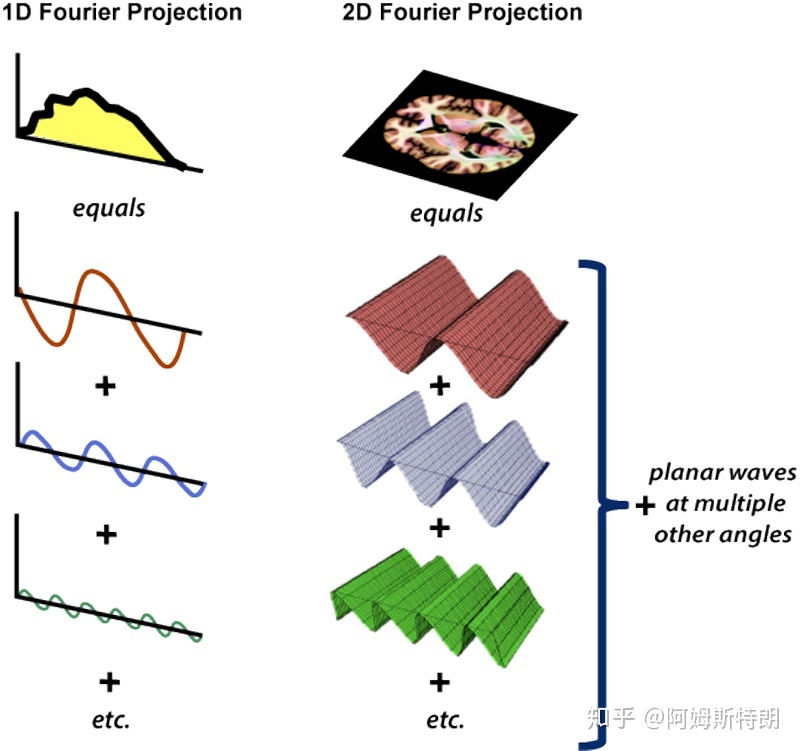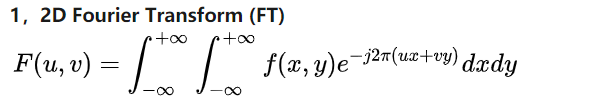二维离散傅里叶变换
二维离散傅里叶变换DFT可分离性的基本思想是DFT可分离为两次一维DFT。因此可以用通过计算两次一维的FFT来得到二维快速傅里叶FFT算法。根据快速傅里叶变换的计算要求，需要图像的行数、列数均满足2的n次方，如果不满足，在计算FFT之前先要对图像补零以满足2的n次。
一个M行N列的二维图像f(x,y)，先按行队列变量y做一次长度为N的一维离散傅里叶变换，再将计算结果按列向对变量x做一次长度为M傅里叶变换就可以得到该图像的傅里叶变换结果，如式所示：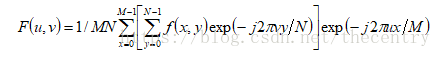将上式分解开来就是如下的两部分，先得到F(x,v),再由F(x,v)得到F(u,v)：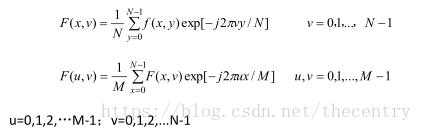每一行由N个点，对每一行的一维N点序列进行离散傅里叶变换得到F(x,u),再对得到F(x,u)按列向对每一列做M点的离散傅里叶变换，就可以得到二维图像f(x,y)的离散傅里叶变换F(u,v).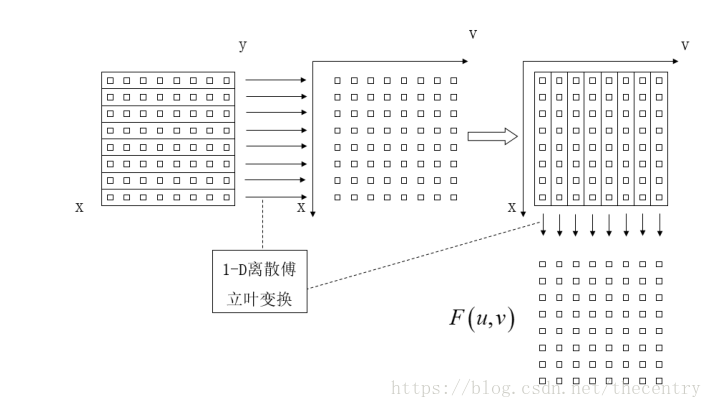同样，做傅里叶逆变换时，先对列向做一维傅里叶逆变换，再对行做一维逆傅里叶变换，如下式所示：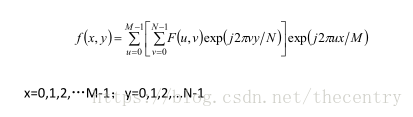展开全文• 实现图像的二维傅里叶变换的频谱图 图像的频谱分析有助于我们理解图像的二维傅里叶变换（离散非周期），并且以直观的方式来展现图像的低通或高通滤波，然而如何获得图像的频谱呢？在matlab中只要短短的几行代码，就...

实现图像的二维傅里叶变换的频谱图

图像的频谱分析有助于我们理解图像的二维傅里叶变换（离散非周期），并且以直观的方式来展现图像的低通或高通滤波，然而如何获得图像的频谱呢？在matlab中只要短短的几行代码，就可以利用库中的函数轻松地做到。

可是，在此过程中都发生了哪些数学变换，以及得到的频谱图的含义，以及二维傅里叶变换的执行过程…都被蒙在了暗盒里。此篇文章将以应用的角度来加深对二维傅里叶变换执行过程的理解。不过，谈不上实际应用，因为普通的二维傅里叶变换实在是…太！慢！了！

由于我只熟悉java，所以用java来写。如果你熟悉的语言不是java并不会影响你对本文的理解，我会一步步解释，保证你用C/C++也能实现它。

1. 图像矩阵f(x,y)装入二维数组

这个简单，在双重循环里获取图像（i，j）坐标处的像素值（这里为了减少运算量，只获取了一个颜色通道），存到二维数组中待下一步操作

for (int i = 0;i<width;i++) {
for(int j=0;j<height;j++){
pixel[i][j]=image.getRGB(i,j)<<24>>24&0xff;
}
}

2.编写二维傅里叶变换类

F ( k , l ) = ∑ x = 0 w i d t h − 1 ∑ y = 0 h e i g h t − 1 f ( x , y ) e − i 2 π ( k x w i d t h + l y h e i g h t ) F(k,l)=\sum_{x=0}^{width-1}\sum_{y=0}^{height-1} f(x,y) e^{-i2\pi(\frac{kx}{width}+\frac{ly}{height})}
由欧拉公式：
e − i x = c o s ( x ) − i s i n ( x ) e^{-ix}=cos(x)-isin(x)
得：
F ( k , l ) = ∑ x = 0 w i d t h − 1 ∑ y = 0 h e i g h t − 1 f ( x , y ) [ c o s ( 2 π ( k x w i d t h + l y h e i g h t ) ) − i s i n ( 2 π ( k x w i d t h + l y h e i g h t ) ) ] F(k,l)=\sum_{x=0}^{width-1}\sum_{y=0}^{height-1} f(x,y)[cos(2\pi(\frac{kx}{width}+\frac{ly}{height}))-isin(2\pi(\frac{kx}{width}+\frac{ly}{height}))]
即最终变换出的结果应该是复数的形式(re指实部，im指虚部)
F ( k , l ) = r e + i ∗ i m F(k,l)=re+i*im
所以在类中定义一个求实部re的方法和求虚部im的方法

static double doDFTre(int pixel[][],int width,int height,int u,int v){
double sum=0;
for(int i=0;i<width;i++){
for(int j=0;j<height;j++){
sum=sum+(pixel[i][j]*pow(-1,i+j)*cos(2*PI*u*i/width+2*PI*v*j/height));
}
}
return sum;
}//Real part
static double doDFTim(int pixel[][],int width,int height,int u,int v){
double sum=0;
for(int i=0;i<width;i++){
for(int j=0;j<height;j++){
sum=sum+(pixel[i][j]*pow(-1,i+j)*sin(2*PI*u*i/width+2*PI*v*j/height));
}
}
return sum;
}//Imaginary part

图像的频谱图中每个像素点的值表示的是以幅值的对数来刻画的（可类比地震级、亮度级）
求幅值Am
A m = r e 2 + i m 2 Am=\sqrt{re^2+im^2}
进行对数变换
方 法 的 返 回 值 是 l g ( 1 + A m ) 2 方法的返回值是lg(1+Am)^2

static int doDFTam(int pixel[][],int width,int height,int i,int j){
re=doDFTre(pixel,width,height,i,j);
});
im=doDFTim(pixel,width,height,i,j);
});
Am=sqrt(pow(re,2)+pow(im,2));
return (int)pow(log(1+Am),2.3)

在多核处理器上，为了计算更快，可以分多个线程并发计算
这里设了两个线程，一个求实部，一个求虚部

3.将低频部分移动到频谱图中心

以上得出的频谱图低频部分分散在四周，高频部分在中央，为了集中低频于中央，可在步骤1中对原图f(i,j)作如下变换得到u(i,j)再对u(i,j)作二维傅里叶变换（这部分我也不理解，只是套公式）
u ( i , j ) = f ( i ， j ) ( − 1 ) i + j u(i,j)=f(i，j)(-1)^{i+j}

4.运行

可以再写一个守护线程用来计时

5.完整的代码

import javax.imageio.ImageIO;
import java.awt.image.BufferedImage;
import java.io.File;
import java.io.IOException;
import static java.lang.Math.*;

public class Fourier2D {
static int logam=0;
public static void main(String[] args) {
int i=0;
while(true){
System.out.println("Time Consumption  "+i+"  sec");
i=i+1;
try {
} catch (InterruptedException e) {
e.printStackTrace();
}
}
});//计时器

try {
int width=image.getWidth();
int height=image.getHeight();

BufferedImage outimage=new BufferedImage(width,height,2);
int pixel[][]=new int[width][height];

for (int i = 0;i<width;i++) {
for(int j=0;j<height;j++){
pixel[i][j]=image.getRGB(i,j)<<24>>24&0xff;
}
}
for(int i=0;i<width;i++){
for(int j=0;j<width;j++){
logam=DFT.doDFTam(pixel,width,height,i,j);
if(logam>255){
logam=255;
}//8-bit png图片亮度等级最大只有255
if(logam<0){
logam=0;
}
outimage.setRGB(i,j,255<<24|logam<<16|logam<<8|logam);
}
}
ImageIO.write(outimage,"png",new File("C:\\Users\\Unicode718A\\Desktop\\学习笔记\\IO\\out.png"));
} catch (IOException e) {
e.printStackTrace();
}
}
}

class DFT{
static double re;
static double im;
static double Am;

static double doDFTre(int pixel[][],int width,int height,int u,int v){
double sum=0;
for(int i=0;i<width;i++){
for(int j=0;j<height;j++){
sum=sum+(pixel[i][j]*pow(-1,i+j)*cos(2*PI*u*i/width+2*PI*v*j/height));
}
}
return sum;
}//Real part
static double doDFTim(int pixel[][],int width,int height,int u,int v){
double sum=0;
for(int i=0;i<width;i++){
for(int j=0;j<height;j++){
sum=sum+(pixel[i][j]*pow(-1,i+j)*sin(2*PI*u*i/width+2*PI*v*j/height));
}
}
return sum;
}//Imaginary part
static int doDFTam(int pixel[][],int width,int height,int i,int j){
re=doDFTre(pixel,width,height,i,j);
});//线程1
im=doDFTim(pixel,width,height,i,j);
});//线程2
try {
} catch (InterruptedException e) {
e.printStackTrace();
}
Am=sqrt(pow(re,2)+pow(im,2));
return (int)pow(log(1+Am),2.3);//Log Transformation of Amplitude
}
}

6.结果展示

100 x 100像素原图对应频谱图256 x 256像素的原图对应频谱图7.普通二维傅里叶变换运算量巨大256 x 256 像素转换的运算时间竟然达到了10分钟，而且还是双线程并发的
而100 x 100像素转换的时间”只要”19秒

为什么会相差…这么巨大？

算算处理器执行循环的总次数就大略明白了：
100 x 100的情况：共2x100 x100 x100 x100=2 x 10^8次
256 x 256的情况：共2x256 x256 x256 x256=8.6 x10^9此
工作量增加了43倍，运算时间也会增加相应的倍数
要是图像再大点，耗时更是无法想象，所以实际应用中的傅里叶变换依赖更高效的算法

以上仅为我个人的理解，如有错误之处，还望大佬们指正。

展开全文傅立叶分析 java 多线程
• 二维傅里叶变换的物理意义 数字图像处理 终于明白二维傅里叶变换是什么意义了数字图像处理
• 实验4 图像的二维傅里叶变换和频谱一、实验目的通过本实验使学生掌握使用MATLAB 进行二维傅里叶变换的方法，加深对二维傅里叶变换的理解和图像频谱的理解。二、实验原理本实验是基于数字图像处理课程中的二维傅里叶...
• 极坐标格式下的二维傅里叶变换与逆变换推导 直角坐标系下的二维傅里叶变换和逆变换分别如下： G(u,v)=∬g(x,y)e−j2π(ux+vy)dxdyg(x,y)=∬G(u,v)ej2π(ux+vy)dudv G(u,v)=\iint g(x,y)e^{-j2\pi (ux+vy)}dxdy \\ g...信号处理 数字信号处理 数学
• 二维傅里叶变换的矩阵表示

千次阅读 2020-03-30 23:25:29
二维傅里叶变换的矩阵表示二维傅里叶变换公式二维傅里叶矩阵公式表达1. F(v,u)F(v,u)F(v,u) 为 R1×1R^{1\times1}R1×1 时2. F(v,u)F(v,u)F(v,u) 为 RNv×MuR^{N_v\times M_u}RNv​×Mu​ 时 说明： 做完了图像处理...matlab 线性代数
• 基于Matlab的二维快速傅里叶函数，将此程序放到同一文件夹内可直接调用！
• 在处理二维矩阵时，常想着如何把时域转换... 傅里叶变换的公式： 拆分到这里，我也很开心的掏出祖传的Matlab写了几行代码来实现： function [Fuv] = Myft2(fxy) [N,M,dim] = size(fxy); if dim > 1 txy = doubmatlab 算法 傅立叶分析
• 用matlab对图像进行二维傅里叶变换

千次阅读 多人点赞 2019-04-27 21:55:16
%进行二维傅里叶变换 F=fftshift(F); %对傅里叶变换后的图像进行象限转换 F=abs(F); %求傅里叶变换的模，我们都知道傅里叶变换后的结果为复数，包含real实部和imag虚部，  %abs就是求复数的模，经过这一步...matlab 二维傅里叶变换
• 二维傅里叶 似模似样的前言 最近的瑕疵检测项目需要在有纹理的产品上做很细致的检测。由于当前做项目使用的还是halcon居多，目前知道的方法还是傅里叶变换比较靠谱。 但仅靠halcon自带的样例并不能很好的理解...二维傅里叶
• 二维傅里叶变换： F(u,v)=∑M=0M−1f(x,y)e−j(ux+vy)2πM，u=0,1,2,⋯ ,M−1F(u,v) = \sum_{M=0}^{M-1} f(x,y) e^{-j (ux+vy) \frac{2\pi} M}， u=0,1,2,\cdots,M-1F(u,v)=M=0∑M−1​f(x,y)e−j(ux+vy)M2π​，u=...
•深度学习...